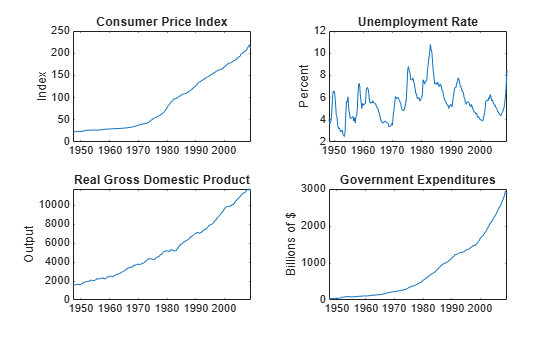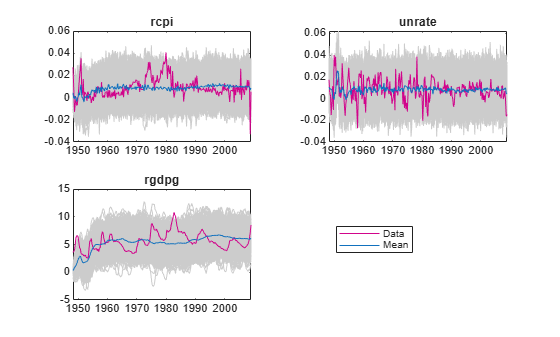Main Content

# filter

Filter disturbances through vector autoregression (VAR) model

## Syntax

``Y = filter(Mdl,Z)``
``Y = filter(Mdl,Z,Name,Value)``
``````[Y,E] = filter(___)``````

## Description

example

````Y = filter(Mdl,Z)` returns the multivariate response series `Y`, which results from filtering the underlying multivariate disturbance series `Z`. The `Z` series are associated with the model innovations process through the fully specified VAR(p) model `Mdl`.```

example

````Y = filter(Mdl,Z,Name,Value)` uses additional options specified by one or more name-value pair arguments. For example, you can specify exogenous predictor data or whether to scale the disturbances by the lower triangular Cholesky factor of the model innovations covariance matrix.```

example

``````[Y,E] = filter(___)``` returns the multivariate model innovations series `E` using any of the input arguments in the previous syntaxes.```

## Examples

collapse all

Fit a VAR(4) model to the consumer price index (CPI) and unemployment rate data. Then, simulate responses by filtering a random series of Gaussian distributed disturbances through the estimated model.

Load the `Data_USEconModel` data set.

`load Data_USEconModel`

Plot the two series on separate plots.

```figure; plot(DataTable.Time,DataTable.CPIAUCSL); title('Consumer Price Index'); ylabel('Index'); xlabel('Date');``````figure; plot(DataTable.Time,DataTable.UNRATE); title('Unemployment rate'); ylabel('Percent'); xlabel('Date');```Stabilize the CPI by converting it to a series of growth rates. Synchronize the two series by removing the first observation from the unemployment rate series. Create a new data set containing the transformed variables, and do not include any rows containing at least one missing observation.

```rcpi = price2ret(DataTable.CPIAUCSL); unrate = DataTable.UNRATE(2:end); idx = all(~ismissing([rcpi unrate]),2); Data = array2timetable([rcpi(idx) unrate(idx)],... 'RowTimes',DataTable.Time(idx),'VariableNames',{'rcpi','unrate'});```

Create a default VAR(4) model using the shorthand syntax.

`Mdl = varm(2,4);`

Estimate the model using the entire data set.

`EstMdl = estimate(Mdl,Data.Variables);`

`EstMdl` is a fully specified, estimated `varm` model object.

Generate a `numobs`-by-2 series of random Gaussian distributed values, where `numobs` is the number of observations in the data.

```numobs = size(Data,1); rng(1) % For reproducibility Z = mvnrnd(zeros(Mdl.NumSeries,1),eye(Mdl.NumSeries),numobs);```

To simulate responses, filter the disturbances through the estimated model.

`Y = filter(EstMdl,Z);`

`Y` is a 245-by-2 matrix of simulated responses. The first and second columns contain the simulated CPI growth rate and unemployment rate, respectively.

Plot the simulated and true responses.

```figure; plot(Data.Time,Y(:,1)); hold on; plot(Data.Time,Data.rcpi) ylabel('Growth rate') xlabel('Date') title('CPI Growth Rate'); legend('Simulation','True')``````figure; plot(Data.Time,Y(:,2)); hold on; plot(Data.Time,Data.unrate) ylabel('Percent'); xlabel('Date') title('Unemployment Rate'); legend('Simulation','True')```Estimate a VAR(4) model of the consumer price index (CPI), the unemployment rate, and the gross domestic product (GDP). Include a linear regression component containing the current quarter and the last four quarters of government consumption expenditures and investment (GCE). Pass multiple multivariate Gaussian disturbance paths through the estimated model.

Load the `Data_USEconModel` data set. Compute the real GDP.

```load Data_USEconModel DataTable.RGDP = DataTable.GDP./DataTable.GDPDEF*100;```

Plot all variables on separate plots.

```figure; subplot(2,2,1) plot(DataTable.Time,DataTable.CPIAUCSL); ylabel('Index'); title('Consumer Price Index'); subplot(2,2,2) plot(DataTable.Time,DataTable.UNRATE); ylabel('Percent'); title('Unemployment Rate'); subplot(2,2,3) plot(DataTable.Time,DataTable.RGDP); ylabel('Output'); title('Real Gross Domestic Product'); subplot(2,2,4) plot(DataTable.Time,DataTable.GCE); ylabel('Billions of \$'); title('Government Expenditures');```Stabilize the CPI, GDP, and GCE by converting each to a series of growth rates. Synchronize the unemployment rate series with the others by removing its first observation.

```inputVariables = {'CPIAUCSL' 'RGDP' 'GCE'}; Data = varfun(@price2ret,DataTable,'InputVariables',inputVariables); Data.Properties.VariableNames = inputVariables; Data.UNRATE = DataTable.UNRATE(2:end);```

Expand the GCE rate series to a matrix that includes its current value and up through four lagged values. Remove the `GCE` variable from `Data`.

```rgcelag4 = lagmatrix(Data.GCE,0:4); Data.GCE = [];```

Create a default VAR(4) model using the shorthand syntax.

```Mdl = varm(3,4); Mdl.SeriesNames = {'rcpi' 'unrate' 'rgdpg'};```

Estimate the model using the entire sample. Specify the GCE matrix as data for the regression component.

`EstMdl = estimate(Mdl,Data.Variables,'X',rgcelag4);`

Generate 1000 paths of `numobs` observations from a 3-D Gaussian distribution. `numobs` is the number of observations in the data without any missing values.

```numpaths = 1000; numseries = Mdl.NumSeries; idx = all(~ismissing([Data array2table(rgcelag4)]),2); numobs = sum(idx); rng(1); Z = mvnrnd(zeros(Mdl.NumSeries,1),eye(Mdl.NumSeries),numobs*numpaths); Z = reshape(Z,[numobs,3,numpaths]);```

Filter the disturbances through the estimated model. Supply the predictor data. Return the innovations (scaled disturbances).

`[Y,E] = filter(EstMdl,Z,'X',rgcelag4);`

`Y` and `E` are 244-by-3-by-1000 matrices of filtered responses and scaled disturbances, respectively. The columns correspond to the CPI growth rate, unemployment rate, and GDP growth rate, respectively. `filter` applies the same predictor data to all paths.

For each time point, compute the mean vector of the filtered responses among all paths.

`MeanFilt = mean(Y,3);`

`MeanFilt` is a 244-by-3 matrix containing the average of the responses at each time point.

Plot the filtered responses, their averages, and the data.

```Data = Data(idx,:); figure; for j = 1:Mdl.NumSeries subplot(2,2,j) plot(Data.Time,squeeze(Y(:,j,:)),'Color',[0.8,0.8,0.8]) title(Mdl.SeriesNames{j}); hold on h1 = plot(Data.Time,Data{:,j}); h2 = plot(Data.Time,MeanFilt(:,j)); hold off end hl = legend([h1 h2],'Data','Mean'); hl.Location = 'none'; hl.Position = [0.6 0.25 hl.Position(3:4)];```## Input Arguments

collapse all

VAR model, specified as a `varm` model object created by `varm` or `estimate`. `Mdl` must be fully specified.

Underlying multivariate disturbance series associated with the model innovations process, specified as a `numobs`-by-`numseries` numeric matrix or a `numobs`-by-`numseries`-by-`numpaths` numeric array.

`numobs` is the sample size. `numseries` is the number of disturbance series (`Mdl.NumSeries`). `numpaths` is the number of disturbance paths.

Rows correspond to sampling times, and the last row contains the latest set of disturbances.

Columns correspond to individual disturbance series for response variables.

Pages correspond to separate, independent paths. For a numeric matrix, `Z` is a single `numseries`-dimensional path of disturbance series. For a 3-D array, each page of `Z` represents a separate `numseries`-dimensional path. Among all pages, disturbances in corresponding rows occur at the same time.

The `'Scale'` name-value pair argument specifies whether to scale the disturbances before `filter` filters them through `Mdl`. For more details, see `Scale`.

Data Types: `double`

### Name-Value Pair Arguments

Specify optional comma-separated pairs of `Name,Value` arguments. `Name` is the argument name and `Value` is the corresponding value. `Name` must appear inside quotes. You can specify several name and value pair arguments in any order as `Name1,Value1,...,NameN,ValueN`.

Example: `'Scale',false,'X',X` does not scale `Z` by the lower triangular Cholesky factor of the model covariance matrix before filtering, and uses the matrix `X` as predictor data in the regression component.

Presample responses that provide initial values for the model `Mdl`, specified as the comma-separated pair consisting of `'Y0'` and a `numpreobs`-by-`numseries` numeric matrix or a `numpreobs`-by-`numseries`-by-`numprepaths` numeric array.

`numpreobs` is the number of presample observations. `numprepaths` is the number of presample response paths.

Rows correspond to presample observations, and the last row contains the latest presample observation. `Y0` must have at least `Mdl.P` rows. If you supply more rows than necessary, `filter` uses the latest `Mdl.P` observations only.

Columns must correspond to the response series in `Y`.

Pages correspond to separate, independent paths.

• If `Y0` is a matrix, then `filter` applies it to each path (page) in `Y`. Therefore, all paths in `Y` derive from common initial conditions.

• Otherwise, `filter` applies `Y0(:,:,j)` to `Y(:,:,j)`. `Y0` must have at least `numpaths` pages, and `filter` uses only the first `numpaths` pages.

Among all pages, observations in a particular row occur at the same time.

By default, `filter` sets any necessary presample observations.

• For stationary VAR processes without regression components, `filter` uses the unconditional mean $\mu ={\Phi }^{-1}\left(L\right)c.$

• For nonstationary processes or models containing a regression component, `filter` sets presample observations to an array composed of zeros.

Data Types: `double`

Predictor data for the regression component in the model, specified as the comma-separated pair consisting of `'X'` and a numeric matrix containing `numpreds` columns.

`numpreds` is the number of predictor variables (`size(Mdl.Beta,2)`).

Rows correspond to observations, and the last row contains the latest observation. `X` must have at least as many observations as `Z`. If you supply more rows than necessary, `filter` uses only the latest observations. `filter` does not use the regression component in the presample period.

Columns correspond to individual predictor variables. All predictor variables are present in the regression component of each response equation.

`filter` applies `X` to each path (page) in `Z`; that is, `X` represents one path of observed predictors.

By default, `filter` excludes the regression component, regardless of its presence in `Mdl`.

Data Types: `double`

Flag indicating whether to scale disturbances by the lower triangular Cholesky factor of the model covariance matrix, specified as the comma-separated pair consisting of `'Scale'` and `true` or `false`.

For each page `j` = 1,...,`numpaths`, `filter` filters the `numobs`-by-`numseries` matrix of innovations `E(:,:,j)` through the VAR(p) model `Mdl`, according to these conditions.

• If `Scale` is `true`, then `E(:,:,j)` = `L*Z(:,:,j)` and `L` = `chol(Mdl.Covariance,'lower')`.

• If `Scale` is `false`, then `E(:,:,j)` = `Z(:,:,j)`.

Example: `'Scale',false`

Data Types: `logical`

Note

`NaN` values in `Z`, `Y0`, and `X` indicate missing values. `filter` removes missing values from the data by list-wise deletion.

1. If `Z` is a 3-D array, then `filter` horizontally concatenates the pages of `Z` to form a `numobs`-by-`numpaths*numseries` matrix.

2. If a regression component is present, then `filter` horizontally concatenates `X` to `Z` to form a `numobs`-by-`(numpaths*numseries + numpreds)` matrix. `filter` assumes that the last rows of each series occur at the same time.

3. `filter` removes any row that contains at least one `NaN` from the concatenated data.

4. `filter` applies steps 1 and 3 to the presample paths in `Y0`.

This process ensures that the filtered responses and innovations of each path are the same size and are based on the same observation times. In the case of missing observations, the results obtained from multiple paths of `Z` can differ from the results obtained from each path individually.

This type of data reduction reduces the effective sample size.

## Output Arguments

collapse all

Filtered multivariate response series, returned as a `numobs`-by-`numseries` numeric matrix or a `numobs`-by-`numseries`-by-`numpaths` numeric array. `Y` represents the continuation of the presample responses in `Y0`.

Multivariate model innovations series, returned as a `numobs`-by-`numseries` numeric matrix or a `numobs`-by-`numseries`-by-`numpaths` numeric array. For details on the value of `E`, see `Scale`.

## Algorithms

• `filter` computes `Y` and `E` using this process for each page `j` in `Z`.

1. If `Scale` is `true`, then `E(:,:,j)` = `L*Z(:,:,j)`, where `L` = `chol(Mdl.Covariance,'lower')`. Otherwise, `E(:,:,j)` = `Z(:,:,j)`. Set et = `E(:,:,j)`.

2. `Y(:,:,j)` is yt in this system of equations.

`${y}_{t}={\stackrel{^}{\Phi }}^{-1}\left(L\right)\left(\stackrel{^}{c}+\stackrel{^}{\delta }t+{e}_{t}\right).$`

For variable definitions, see More About.

• `filter` generalizes `simulate`. Both functions filter a disturbance series through a model to produce responses and innovations. However, whereas `simulate` generates a series of mean-zero, unit-variance, independent Gaussian disturbances `Z` to form innovations `E` = `L*Z`, `filter` enables you to supply disturbances from any distribution.

• `filter` uses this process to determine the time origin t0 of models that include linear time trends.

• If you do not specify `Y0`, then t0 = 0.

• Otherwise, `filter` sets t0 to `size(Y0,1)``Mdl.P`. Therefore, the times in the trend component are t = t0 + 1, t0 + 2,..., t0 + `numobs`, where `numobs` is the effective sample size (`size(Y,1)` after `filter` removes missing values). This convention is consistent with the default behavior of model estimation in which `estimate` removes the first `Mdl.P` responses, reducing the effective sample size. Although `filter` explicitly uses the first `Mdl.P` presample responses in `Y0` to initialize the model, the total number of observations in `Y0` and `Y` (excluding missing values) determines t0.

 Hamilton, James D. Time Series Analysis. Princeton, NJ: Princeton University Press, 1994.

 Johansen, S. Likelihood-Based Inference in Cointegrated Vector Autoregressive Models. Oxford: Oxford University Press, 1995.

 Juselius, K. The Cointegrated VAR Model. Oxford: Oxford University Press, 2006.

 Lütkepohl, H. New Introduction to Multiple Time Series Analysis. Berlin: Springer, 2005.

Download ebook